## Tuesday, September 19, 2017

### Statistical properties of `posthoc` method for `"ratebytree"` object class

Yesterday, I wrote about a new post-hoc test for `ratebytree`. Today, I thought I would do a few additional things with this as follows:

1) Demonstrate how the method can be used to compare parameters of an OU (or EB) process between trees.

2) Examine the type I error of the method using no or various different corrections to take into account multiple testing.

3) Finally, compare the P-values obtained via likelihood-ratio to those from the posthoc test when only two trees are compared (they should be the same or similar).

Note that I have made some small updates to `ratebytree` for this exercise. Details of these can be seen here.

First (1).

Here I'll use the following trees & data:

``````library(phytools)
packageVersion("phytools")
``````
``````##  '0.6.27'
``````
``````print(trees,details=TRUE)
``````
``````## 3 phylogenetic trees
## tree 1 : 40 tips
## tree 2 : 50 tips
## tree 3 : 60 tips
``````
``````par(mfrow=c(1,3))
## trait x
for(i in 1:length(trees))
phenogram(trees[[i]],x[[i]],
ylim=range(x),label.pos=setNames(
seq(min(sapply(x,min)),
max(sapply(x,max)),
diff(range(x))/(Ntip(trees[[i]])-1)),
names(sort(x[[i]]))),
col=make.transparent("blue",0.5))
``````
``````## Optimizing the positions of the tip labels...
``````
``````## Optimizing the positions of the tip labels...
``````
``````## Optimizing the positions of the tip labels...
``````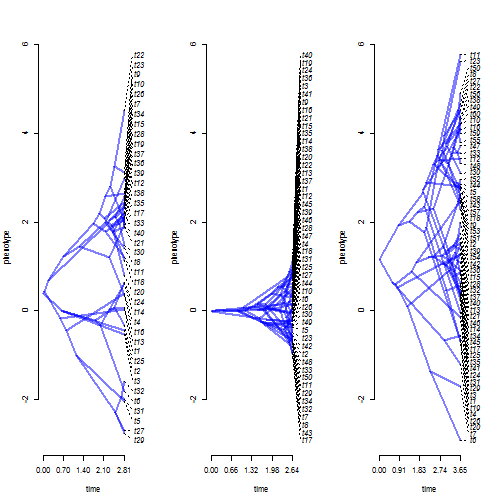``````## trait y
for(i in 1:length(trees))
phenogram(trees[[i]],y[[i]],
ylim=range(y),label.pos=setNames(
seq(min(sapply(y,min)),
max(sapply(y,max)),
diff(range(y))/(Ntip(trees[[i]])-1)),
names(sort(y[[i]]))),
col=make.transparent("blue",0.5))
``````
``````## Optimizing the positions of the tip labels...
``````
``````## Optimizing the positions of the tip labels...
``````
``````## Optimizing the positions of the tip labels...
``````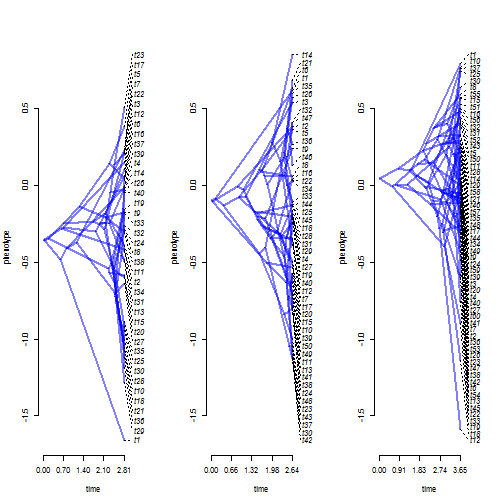In trait x, it does indeed look like tree 2 might be evolving by a different (OU?) process than the other clades, but in trait y it is harder to tell.

Let's fit our models as we did yesterday:

``````fitOU.x<-ratebytree(trees,x,model="OU")
fitOU.x
``````
``````## ML common-regime OU model:
##          s^2  a   a    a    alpha   k   logL
## value    1.0524  0.3973  -0.0157 1.1755  0.0448  5   -200.5537
## SE       0.1749  0.5885  0.5979  0.7325  0.107
##
## ML multi-regime OU model:
##          s^2 s^2  s^2   a   a    a     alp alp  alp  k   logL
## value    1.1236  1.3133  1.3639  0.3985  0.0032  1.1635  0.0744  2.0296  -0.1692 9   -185.2541
## SE       0.3389  0.5097  0.249   0.5759  0.1011  0.9402  0.1908  0.8769  0
##
## Likelihood ratio: 30.5992
## P-value (based on X^2): 0
##
## R thinks it has found the ML solution.
``````
``````posthoc(fitOU.x)
``````
``````##
## Post-hoc test for "OU" model.
## (Comparison is of estimated values of alpha.)
##
##                   t      df      P
## tree1 vs. 2 -3.1942 81.6326 0.0020
## tree1 vs. 3  0.5792 63.1136 0.5645
## tree2 vs. 3  3.8760 65.4179 0.0002
##
``````
``````fitOU.y<-ratebytree(trees,y,model="OU")
fitOU.y
``````
``````## ML common-regime OU model:
##          s^2  a   a    a    alpha   k   logL
## value    0.8042  -0.3228 -0.1424 0.0602  1.5824  5   -92.6983
## SE       0.193   0.1064  0.0958  0.0795  0.4328
##
## ML multi-regime OU model:
##          s^2 s^2  s^2   a   a    a     alp alp  alp  k   logL
## value    0.9544  0.7473  0.7745  -0.3248 -0.1411 0.0596  1.8196  1.3618  1.6621  9   -92.4482
## SE       0.4514  0.2639  0.361   0.1045  0.1048  0.0755  0.9626  0.5738  0.8639
##
## Likelihood ratio: 0.5003
## P-value (based on X^2): 0.9735
##
## R thinks it has found the ML solution.
``````
``````posthoc(fitOU.y)
``````
``````##
## Post-hoc test for "OU" model.
## (Comparison is of estimated values of alpha.)
##
##                   t       df      P
## tree1 vs. 2  0.8756  56.6571 0.3850
## tree1 vs. 3  0.2725  67.3268 0.7861
## tree2 vs. 3 -0.6717 102.4482 0.5033
##
``````

The posthoc test only compares the values of the fitted model parameters: α for the OU model, r for the EB/ACDC model, and so on.

Our results show that in trait x there is a difference in process between trees. Trait x seems to have evolved by an OU process with a reasonably high value of α in tree 2, but low values of α (or perhaps by a BM process, which corresponds to α = 0) on trees 1 & 3.

In trait y there is no evidence for a difference in process between trees.

This is pretty good because it closely matches the conditions that we simulated, as follows:

``````trees<-c(pbtree(n=40),pbtree(n=50),pbtree(n=60))
class(trees)<-"multiPhylo"
x<-list(fastBM(trees[]),fastBM(trees[],model="OU",alpha=2),
fastBM(trees[]))
y<-list(fastBM(trees[],model="OU",alpha=1.5),
fastBM(trees[],model="OU",alpha=1.5),
fastBM(trees[],model="OU",alpha=1.5))
``````

Next, (2), I'm going to conduct a very simple type I error test of our posthoc method & test whether the adjustment methods for multiple testing result in the correct experiment-wise type I error rate. To do this, I will simulate by Brownian evolution on four trees with a constant rate, and from each analysis I will extract four values: first, the P-value for the whole test; & second, the number of times any P-value for our posthoc test was < 0.05 using 3 different corrections - `"none"` (no correction), `"bonferroni"`, and `"BH"` (for more details see the documentation page for `stats::p.adjust`.

Here I go:

``````## first, simulate the trees & data
nrep<-200
tt<-replicate(nrep,lapply(c(40,50,60,70),function(n) pbtree(n=n)),simplify=FALSE)
tt<-lapply(tt,function(x){ class(x)<-"multiPhylo"; x})
X<-lapply(tt,function(tt) lapply(tt,fastBM))
## now fit our models
fitBMs<-mapply(ratebytree,trees=tt,x=X,SIMPLIFY=FALSE)
none<-lapply(fitBMs,posthoc)
## get our P values or error rates
P.full<-sapply(fitBMs,function(x) x\$P.chisq)
typeI.none<-mean(sapply(none,function(x) any(x\$P<=0.05)))
typeI.bonferroni<-mean(sapply(bonferroni,function(x) any(x\$P<=0.05)))
typeI.BH<-mean(sapply(BH,function(x) any(x\$P<=0.05)))
## visualize our P-values for the full test
obj<-hist(P.full,breaks=seq(0,1,by=0.05),plot=F)
plot(obj\$mids,obj\$counts/sum(obj\$counts),type="h",
lwd=18,col=make.transparent("blue",0.4),lend=1,
xlab="P-value",ylab="relative frequency",ylim=c(0,0.2))
title(main="P-values for full test from data simulated under the null")
abline(h=0.05,col=make.transparent("red",0.5),lwd=1,
lty="dotted")
text(0.96,0.05,"0.05",pos=3)
``````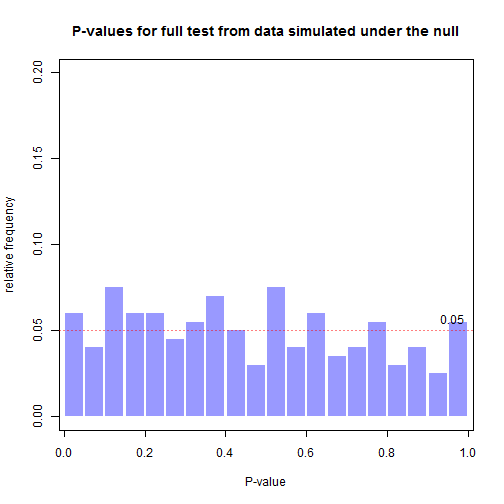``````## visualize our experiment-wise type I error rates for each p.adjust.method
library(plotrix)
typeI<-setNames(c(typeI.none,typeI.bonferroni,typeI.BH),c("none","bonferroni","BH"))
plotCI(x=c(1,2,3),y=typeI,uiw=sqrt(typeI*(1-typeI)/nrep),pch=21,pt.bg="grey",
cex=2,xlab="correction method",ylab="experiment-wise type I error rate",
xaxt="n",xlim=c(0.5,3.5),ylim=c(0,2*max(typeI)),
main="experiment-wise type I error rate from posthoc tests")
axis(1,1:3,labels=FALSE)
mtext(names(typeI),at=1:3,side=1,line=1)
abline(h=0.05,col="red",lty="dotted")
text(3.45,0.05,"0.05",pos=3)
``````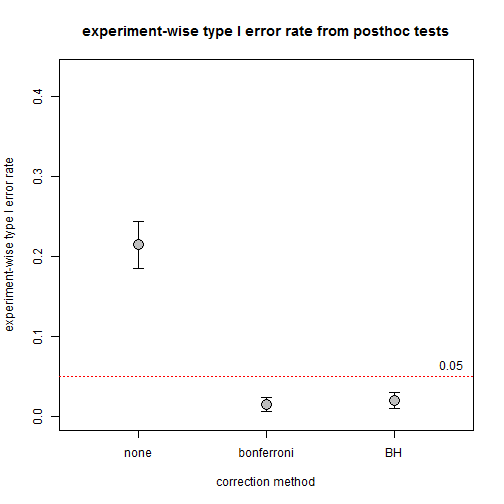This should show as that no correction results in an elevated experiment-wise type I eror rate; and perhaps that `"bonferroni"` is excessively corrective and `"BH"` in between. (At least that is what the documentation of `p.adjust` would suggest.)

Finally, (3), let's compare the posthoc P-values to the P-values from our likelihood ratio test when we only have two trees. Like a t-test and an ANOVA for two groups - these should match. (Although, unlike the ANOVA and t-test the calculation differs - the t-values are computed from SEs obtained from the Hessian, while our likelihood ratio is computed from the difference in log-likelihood between two models - so I do not know whether or not theory informs us that they need be identical as between an ANOVA with two levels & a t-test.)

Let's investigate:

``````## create vectors in which to store our results
P.t<-P.lr<-vector()
tt<-replicate(nrep,lapply(rep(60,2),function(n) pbtree(n=n)),simplify=FALSE)
tt<-lapply(tt,function(x){ class(x)<-"multiPhylo"; x})
X<-lapply(tt,function(tt) lapply(tt,fastBM))
fitBMs<-mapply(ratebytree,trees=tt,x=X,SIMPLIFY=FALSE)
P.lr<-sapply(fitBMs,function(x) x\$P.chisq)
P.t<-sapply(fitBMs,function(x) posthoc(x)\$P[1,2])
plot(P.lr,P.t,cex=1.6,bg="grey",pch=21,xlab="P-values based on likelihood-ratio",
ylab="P-values from posthoc t-tests")
``````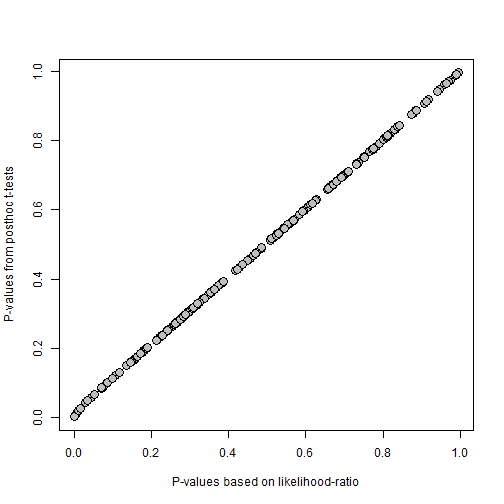That's my three objectives done. Neat.